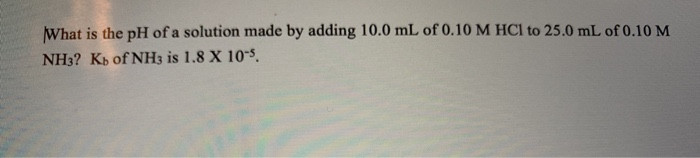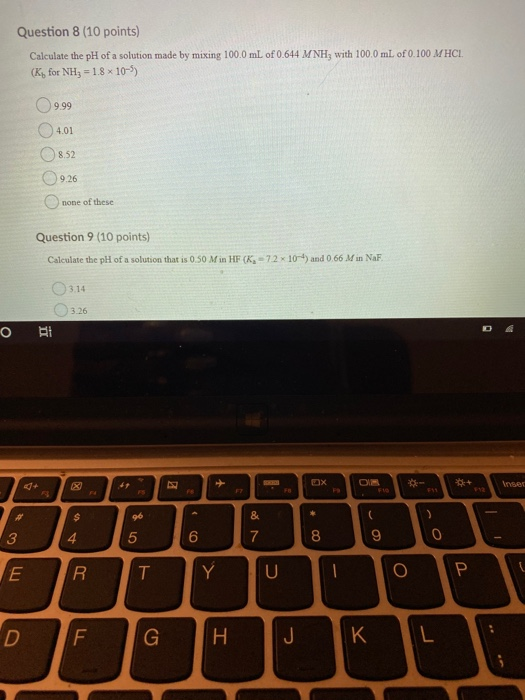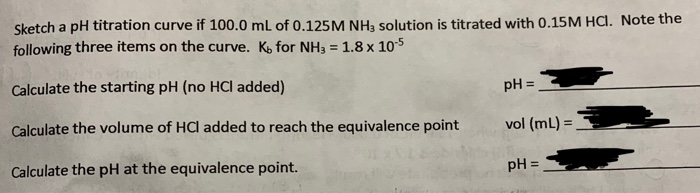Question

(1). The pH of a solution made by mixing 100.0 mL of 0.433 M NH3 with 100.0 mL of 0.259 M HCl is ___.  Kb = 1.8 x 10-5 for NH3.

We need at least 10 more requests to produce the answer.

0 / 10 have requested this problem solution

The more requests, the faster the answer.

All students who have requested the answer will be notified once they are available.

#### Earn Coins

Coins can be redeemed for fabulous gifts.

Similar Homework Help Questions
• ### Calculate the pH of a solution made by mixing 100.0 mL of 0.874 M NH3 with...

Calculate the pH of a solution made by mixing 100.0 mL of 0.874 M NH3 with 100.0 mL of 0.100 M HCl. (Kb for NH3 = 1.8 10

• ### Calculate the pH of a solution made by mixing 100.0 mL of 0.355 M NH3 with...

Calculate the pH of a solution made by mixing 100.0 mL of 0.355 M NH3 with 100.0 mL of 0.100 M HCl. (Kb for NH3 = 1.8 10–5) Select one: a. 9.66 b. 8.85 c. 4.34 d. 9.26

• ### Calculate the pH of a solution obtained by mixing 25.00 mL of 0.13 M NH3 with...

Calculate the pH of a solution obtained by mixing 25.00 mL of 0.13 M NH3 with 25.00 mL of 5.0×10-2 M HCl. Kb for NH3 is 1.8×10-5.

• ### What is the pH of a solution prepared by mixing 50.00 mL of 0.10 M NH3...

What is the pH of a solution prepared by mixing 50.00 mL of 0.10 M NH3 with 5.00 mL of 0.10 M NH4Cl? Assume that the volume of the solutions are additive and that Kb = 1.8 × 10-5 for NH3. (Select One) 10.26 or 11.13

• ### What is the pH of a solution made by adding 10.0 mL of 0.10 M HCl...What is the pH of a solution made by adding 10.0 mL of 0.10 M HCl to 25.0 mL of 0.10 M NH3? Kb of NH3 is 1.8 X 10-5.

• ### 1) Calculate the pH of a solution produced by mixing 100.0 mL of 0.10 M HCl(aq)...

1) Calculate the pH of a solution produced by mixing 100.0 mL of 0.10 M HCl(aq) and 100.0 mL of 0.20 M NaOH(aq). 2) Calculate the pH of an aqueous solution made by mixing 100.0 mL of 0.40 M NH4Cl and 50.0 mL of 0.40 M NaOH. The pKa of NH4+ is 9.24.

• ### Question 8 (10 points) Calculate the pH of a solution made by mixing 100.0 mL of...Question 8 (10 points) Calculate the pH of a solution made by mixing 100.0 mL of 0.644 MNH; with 100.0 ml. of 0.100 MHCI. (K for NH; = 1.8 x 10-) 9.99 4.01 8.52 9.26 none of these Question 9 (10 points) Calculate the pH of a solution that is 0.50 Min HF (K-72 x 10-) and 0.66 Min NaF 3.14 3.26 b BE

• ### a) What is the pH of the solution that results from mixing 50 mL of 0.032...

a) What is the pH of the solution that results from mixing 50 mL of 0.032 M NH3 and 50 mL of 0.032 M HCl? (Kb for ammonia is 1.8 x 10-5) b) The compound Xe(CF3)2 decomposes in a first-order reaction to elemental Xe with a half-life of 20 minutes. If you place 15 mg of Xe(CF3)2 in a flask, how long will it take until only 0.5 mg of Xe(CF3)2 remains? c) What indicator would be most suitable for...

• ### Sketch a pH titration curve if 100.0 mL of 0.125 M NH3 solution is titrated with...Sketch a pH titration curve if 100.0 mL of 0.125 M NH3 solution is titrated with 0.15M HCl. Note the following three items on the curve. K, for NHa 1.8 x 105 pH= Calculate the starting pH (no HCl added) vol (mL)= Calculate the volume of HCl added to reach the equivalence point pH = Calculate the pH at the equivalence point.

• ### Calculate the pH of a buffer made from mixing 7.6 mL of 0.259 M NaC2H3O2 and...

Calculate the pH of a buffer made from mixing 7.6 mL of 0.259 M NaC2H3O2 and 12.1 mL of 0.338 M HC2H3O2. The Ka of HC2H3O2 is 1.8 x 10-5.